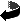# The first conformal Dirac eigenvalue on 2-dimensional tori by Bernd Ammann, Emmanuel Humbert

The first conformal Dirac eigenvalue on 2-dimensional tori (.dvi, .ps,.ps.gz or .pdf)
J. Geom. Phys. 56, 623-642 (2006)

### Abstract

Let M be a compact manifold with a spin structure \chi and a Riemannian metric g. Let \lambda_g^2 be the smallest eigenvalue of the square of the Dirac operator with respect to g and \chi. The \tau-invariant is defined as
\tau(M,\chi):= sup inf \sqrt{\lambda_g^2} Vol(M,g)^{1/n}
where the supremum runs over the set of all conformal classes on M, and where the infimum runs over all metrics in the given class.
We show that \tau(T^2,\chi)=2\sqrt{\pi} if \chi is the'' non-trivial spin structure on T^2. In order to calculate this invariant, we study the infimum as a function on the spin-conformal moduli space and we show that the infimum converges to 2\sqrt{\pi} at one end of the spin-conformal moduli space.Zurück zur Homepage

The Paper was written on 20.12.2004
Last update 20.12.2004